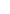## Circuit simulation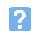1. What does the acronym SPICE stand for?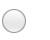a) Simulation Program for Internal Circuit Evaluationb) Simulation Program and Integrated Component Editorc) Standard Program for Integrated Circuit Evaluationd) Simulation Program with Integrated Circuit Emphasis.2. During a DC circuit analysis:a) all inductors and capacitors are replaced by open-circuitsb) all inductors and capacitors are replaced by short-circuitsc) inductors are made open-circuit and capacitors made short-circuitd) inductors are made short-circuit and capacitors made open-circuit.3. A DC circuit analysis is usually performed:a) after a transient analysisb) after a pole-zero analysisc) prior to a transient or pole-zero analysisd) at the same time as performing a transient analysis.4. The transconductance of a circuit is obtained by:a) dividing the output current by the input voltageb) dividing the input current by the output voltagec) dividing the output voltage by the input currentd) dividing the output current by the output voltage.5. The circuit undergoing SPICE analysis in the example shown below is: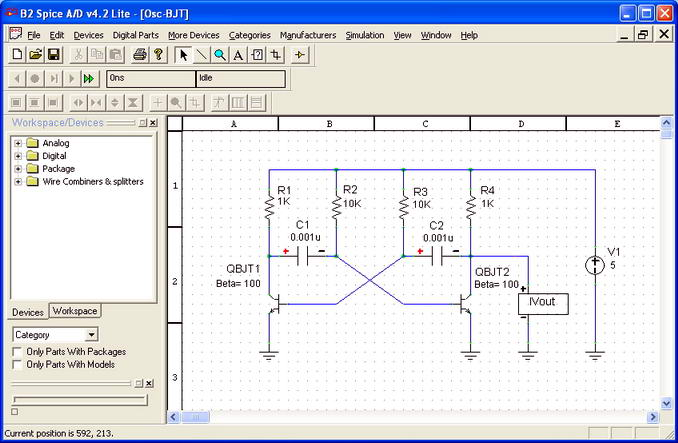a) a monostable timerb) a two-stage amplifierc) a sinusoidal oscillatord) an astable multivibrator.6. The transient analysis shown below has been used to produce: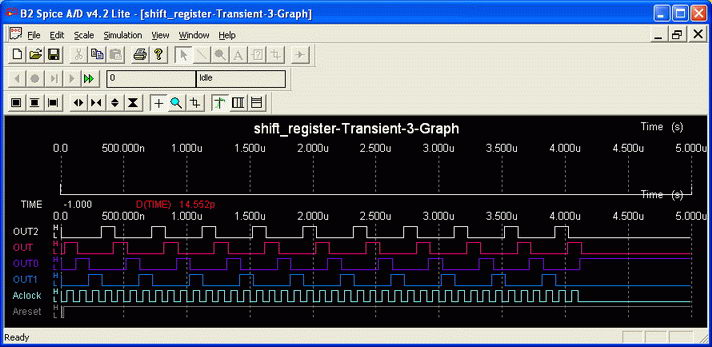a) a timing diagramb) a pole-zero analysisc) a distortion analysisd) a sensitivity analysis.7. Hardware description language (HDL) provides a means of accurately modelling the performance of a complex logic arrangement by using:a) accurate truth tables for each deviceb) Boolean algbra to solve logic functionsc) a standard library of pin connecting datad) a formal and rigorous description of the logic.8. When p and q are both std_ulogic_vector VHDL data types with values 1010 and 0110, respectively the expression "p AND NOT q" evaluates:a) 0001b) 1100c) 1011d) 1000.
This is more feedback!
This is the feedback!